Courses

# Test: Physical & General Properties Of Alkyl Halides

## 22 Questions MCQ Test Chemistry for JEE | Test: Physical & General Properties Of Alkyl Halides

Description
This mock test of Test: Physical & General Properties Of Alkyl Halides for Class 12 helps you for every Class 12 entrance exam. This contains 22 Multiple Choice Questions for Class 12 Test: Physical & General Properties Of Alkyl Halides (mcq) to study with solutions a complete question bank. The solved questions answers in this Test: Physical & General Properties Of Alkyl Halides quiz give you a good mix of easy questions and tough questions. Class 12 students definitely take this Test: Physical & General Properties Of Alkyl Halides exercise for a better result in the exam. You can find other Test: Physical & General Properties Of Alkyl Halides extra questions, long questions & short questions for Class 12 on EduRev as well by searching above.
QUESTION: 1

### Only One Option Correct Type Direction (Q. Nos. 1-9) This section contains 9 multiple choice questions. Each question has four choices (a), (b), (c) and (d), out of which ONLY ONE option is correct. Q.  What is the major product of the reaction?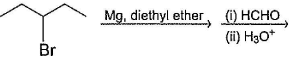Solution: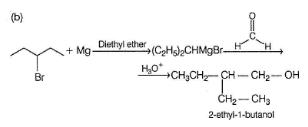QUESTION: 2

### Which are the products of the following sequence of reaction?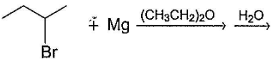Solution: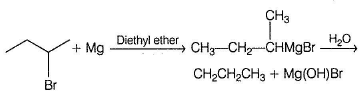QUESTION: 3

### What is the product of the reaction shown?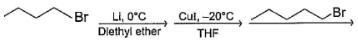Solution: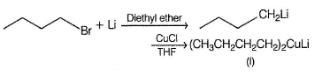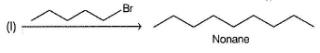QUESTION: 4

What set of reagents can be used to make octane from butyl chloride?

Solution: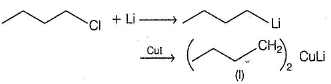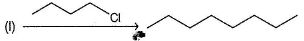QUESTION: 5

Which of the following synthesis give 3-methyl-1 -hexanol?

Solution: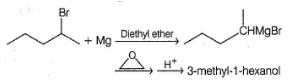QUESTION: 6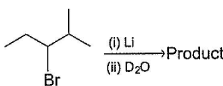Q.

What is the product in the above reaction?

Solution: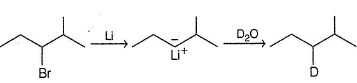QUESTION: 7

Chlorinated hydrocarbon

Solution:

All of these are true regarding chlorinated hydrocarbons.

QUESTION: 8

Which of the following alkyl halides cannot be converted into Grignard reagents?

Solution:

Halides contain ing acidic hydrogen (here — OH) cannot be used in Grignard's synthesis as Grignard reagent consist of very basic carbanion (R-).

QUESTION: 9

Which one is liquid at room temperature?

Solution:

All others are gas at room temperature.

*Multiple options can be correct
QUESTION: 10

One or More than One Options Correct Type

Direction (Q. Nos. 10-14) This section contains 5 multiple choice questions. Each question has four choices (a), (b), (c) and (d), out of which ONE or MORE THAN ONE are correct.

Q.

1-chlorobutane can be reduced to butane by

Solution:

1 -chlorobutane is a primary halide, LiAIH4, Zn, (C6 H5)3SnH all reduces it to butane but NaBH4 does not reduce primary halide.

*Multiple options can be correct
QUESTION: 11

Tertiary butyl chloride can be reduced to 2-methyl propane by

Solution:

LiAIH4 fails to reduce tertiary halide, rather elimination reaction takes place giving alkene.

*Multiple options can be correct
QUESTION: 12

Which of the following float over water?

Solution:

Chloro and fluoro alkanes are less dense than water while bromo and iodoalkane are more denser than water.

*Multiple options can be correct
QUESTION: 13

Which of the following halides are not suitable for Grignard’s synthesis?

Solution:

Both contain acidic hydrogen, not suitable for preparation of Grignard reagent.

*Multiple options can be correct
QUESTION: 14

What is the expected product in the following reaction?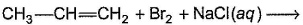Solution:

Cyclic brom onium ion formed in the first step un dergo nucleophilic attack by both Br- and Cl- ions, giving both option (b) and option (c).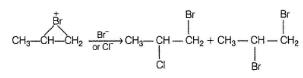QUESTION: 15

Comprehension Type

Direction (Q. Nos. 15-17) This section contains a paragraph, describing theory, experiments, data, etc. Three questions related to the paragraph have been given. Each question has only one correct answer among the four given options (a), (b), (c) and (d).

Passage

In alkyl halides C—X bond is polar. Polarity of C—X bond is responsible for several characteristic reactions of alkyl halides viz.

R—X + H- (from metal hydride) → R —H + X-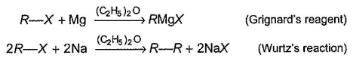Q.

Which of the following will most easily be reduced by LiAIH4?

Solution:

As shown in mechanism, reduction occur by hydride transfer (SN2) mechanism. Hence, primary halide would be most reactive here.

QUESTION: 16

Passage

In alkyl halides C—X bond is polar. Polarity of C—X bond is responsible for several characteristic reactions of alkyl halides viz.

R—X + H- (from metal hydride) → R —H + X-Q.

Which forms Grignard’s reagent with maximum difficulty?

Solution:

Grignard's reagent contain alkyl carbanion (R-). Hence, Grignard's reagent with 3° carbanions are very less likely to be formed.

QUESTION: 17

Passage

In alkyl halides C—X bond is polar. Polarity of C—X bond is responsible for several characteristic reactions of alkyl halides viz.

R—X + H- (from metal hydride) → R —H + X-Q.

Which of the following required more than one type of alkyl halides for its preparation by Wurtz’s reaction?

Solution:

If two similar alkyl halides join by Wurtz’s reaction alkane containing even number of carbons are obtained. If mixed alkyl halides with one containing even and other containing odd number of carbons are used, alkane with odd number of carbons are obtained.

*Answer can only contain numeric values
QUESTION: 18

One Integer Value Correct Type

Direction (Q. Nos. 18-20) This section contains 3 questions. When worked out will result in an integer from 0 to 9 (both inclusive).

Q.

Consider the following reactions,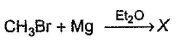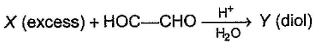Q.

How many different diols are formed?

Solution: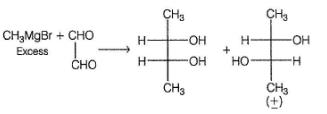*Answer can only contain numeric values
QUESTION: 19

Dichloroalkanes with appropriate skeleton, on refluxing with Na in diethyi ether undergo cyclisation via Wurtz’s reaction. How many different isomeric dibromoalkanes on refluxing with Na metal in diethyl ether can give the same 1,4-dimethyl cyclohexane?

Solution: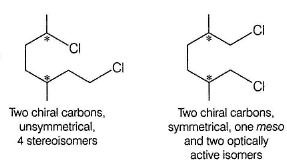Both of the above dichloride on heating with aikali metal gives the same 1,4-dimethyl cyclohexane.

*Answer can only contain numeric values
QUESTION: 20

How many of the following are denser than water?
i. CCl
ii. CH3CH2CH2CH2CI
iii. Bromocyclohexane
iv. Chiorocyclopentane
v. 1 ,3-difiuorocyclopentane
vi. CH3I
vii. 1-fluorodecane
viti. CH2Br2
ix. CH3CI
X. CHCl3

Solution:

Only (ii), (iv), (vii) and (ix) are less dense than water. Alkyl bromides, alkyl iodides and all alkyl halides containing two or more halogen atoms.

QUESTION: 21

Statement Type

Direction (Q. Nos. 21 and 22) This section is based on Statement I and Statement II. Select the correct answer from the codes given below.

Q.

Statement I : CH3F is less polar than CH3CI.

Statement II : F has greater electronegativity than Cl.

Solution:

Although F is more electronegative than Cl, CH3F, is less polar. Dipole moment (μ) is defined as
μ = q.d
Due to smaller radius of F than Cl, q .d in CH3CI is greater than in CH3F although q is greater in CH3F.

QUESTION: 22

Statement I : A tertiary halide (R3CX) is very less likely to form Grignard’s reagent with Mg in THF.

Statement II : Tertiary carbanion are highly unstable, very less likely to be formed.

Solution:

Tertiary carbanion is very unstable, difficult to form.